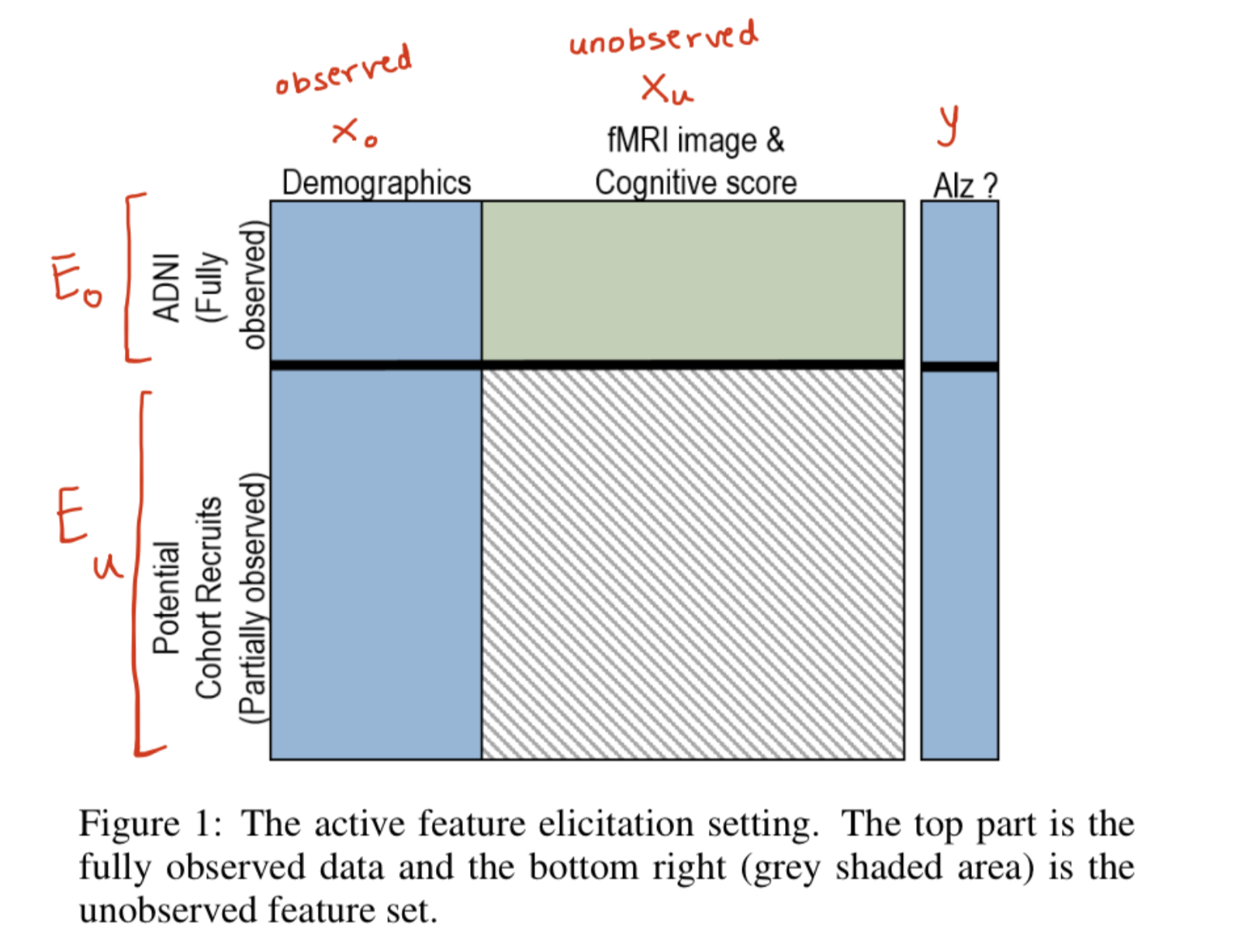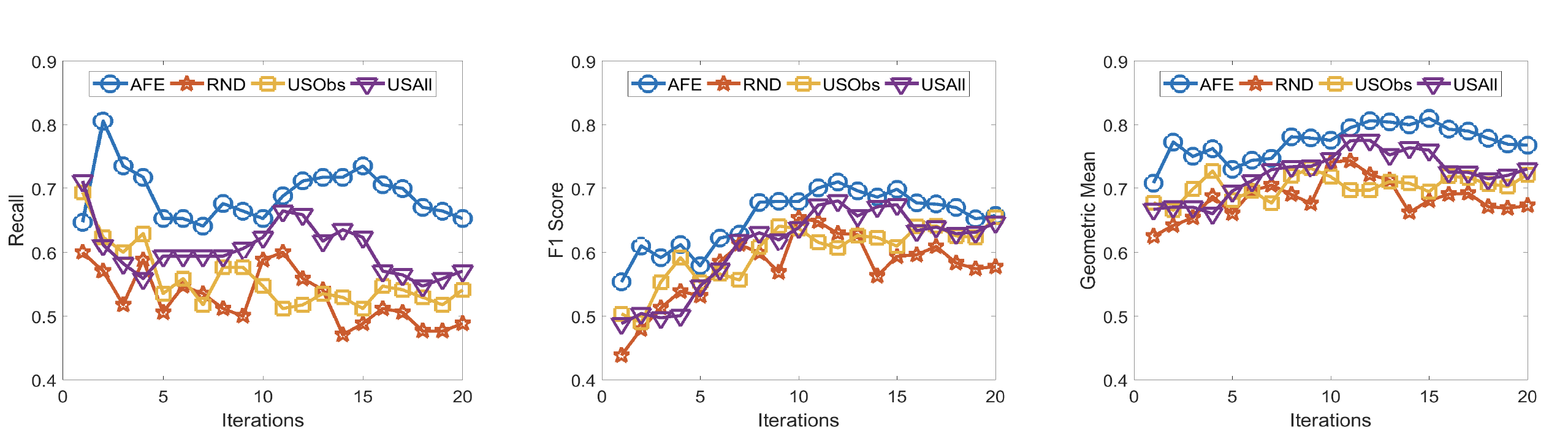## Active Feature Elicitation

Natarjan et al., IJCAI 2018 is one of the most ebullient papers from the Starling Lab (in my opinion, of course!). It formalizes a unique problem setting called Active Feature Elicitation. The task here is to select the best set of examples on whom the missing features can be queried actively. This blog post summarizes my understanding of that paper.

### Motivation

For the success of clinical studies, it is important to recruit people with diverse features. Not all the features are readily available when the decision about recruitment is done. Some features such as demographic details are available at no additional cost while other details like the MRI Image which are costly can be elicitated if the patient is recruited. But since these costly features are not already available during the decision of recruitment, we need a principled approach to make the decision of recruitment in the absence of costly features.

### Objective

The objective of this paper is to define the active feature elicitation problem and provide an approach to this problem.

Active feature elicitation is the problem setting where the goal is to select the best set of examples on whom the missing features can be queried on to best improve the classifier performance.The top part shows the part of the data that is fully observed ($\mathbf{E}_{o}$). The bottom left quadrant shows the observed features($\mathbf{X}_{o}$) of the potential cohorts and the right quadrant is the data that needs to be collected ($\mathbf{X}_{u}$)for the most useful potential recruits. Given, the labels of the potential recruits ($y$), the goal is to identify the most informative cohorts that would aid the study($\mathbf{E}_{a} \subset \mathbf{E}_{u}$).

### Summary

First, let us look at some baseline approaches.

Random ($RND$): The most common approach to recruit cohort from potential candidates is to randomly pick the patients.

Uncertainty Sampling:

a) observed feature only ($USobs$):
An informed approach is to look at all the observed features – $\mathbf{X}_{o}$ for all the examples $\mathbf{E} = \mathbf{E}_{o} \cup \mathbf{E}_{u}$ and learn a classifier that predicts the label ($y$) given only observed features. Now, the potient recruitment subset $\mathbf{E}_{a}$ can be obtained by picking $n$ most uncertain examples from this classifier.

b) all features ($USAll$): More informed approach will be to impute the unobserved features – $\mathbf{X}_{u}$ using mean, mode or some strategy, learn a classifier and sample the subset $\mathbf{E}_{a}$ which has maximum prediction uncertainty.

Sampling based on the maximum prediction uncertainty means we recruit the candidates who have the highest entropy i.e. probability distribution [0.5,0.5] for binary classification.

Although this makes sense in an active learning setting, where we want to obtain a label for example which has maximum uncertainty. In the current setting of active feature elicitation, where we want to recruit the most diverse cohort, the entropy does not make sense. The authors argue that we should look for the most diverse prediction distribution and propose the following approach.

First, build two classifiers:

1. $M_o = P(y | \mathbf{X}_{o})$ which predicts class label based on only the observed features . Train it on all the examples $\mathbf{E} = \mathbf{E}_{o} \cup \mathbf{E}_{u}$.

2. $M_u = P(y | \mathbf{X}_{o}, \mathbf{X}_{u})$ which predicts class label based on all the features. Train it only on example set $\mathbf{E}_{o}$

Since both the models are trained to achieve same prediction for the set $\mathbf{E}_{o}$, we assume that the probability distribution of both the model is similar of set $\mathbf{E}_{o}$.

$$P\left(y^{j} | \mathbf{X}_{o}^{j}, \mathbf{X}_{u}^{j}\right) \approx P\left(y^{j} | \mathbf{X}_{o}^{j}\right) \quad \forall i \in \mathbf{E}_{o}$$

Now, we want to recruit candidates from set $\mathbf{E}_{u}$ which are different than the existing candidate set $\mathbf{E}_{o}$. If we use the probability distribution $P(y_j | \mathbf{X}_{o}^j, \mathbf{X}_{u}^j)$ as a representative of each example in the set $\mathbf{E}_{o}$, we want to find example $i$ which is at maximum distance from $\mathbf{E}_{o}$. Since, we do not have $P(y_i | \mathbf{X}_{o}^i, \mathbf{X}_{u}^i)$, authors propose to use $P(y_i | \mathbf{X}_{o}^i)$ to represent examples from set $\mathbf{E}_{u}$

Now, we can use any divergence/distance measure to compute the farthest example. For example, if we use the KL divergence,

$$D_{i j}=\mathrm{KL}\left(\mathrm{P}\left(y^{i} | \mathbf{X}_{o}^{i}\right) | \mathrm{P}\left(y^{j} | \mathbf{X}_{o}^{j}, \mathbf{X}_{u}^{j}\right)\right)$$

we can compute the mean distance for each example $i \in \mathbf{E}_{u}$:

$$\mathrm{MD}_{i}=\frac{1}{\left|\mathbf{E}_{o}\right|} \sum_{j=1}^{\left|\mathbf{E}_{o}\right|} D_{i j}$$

and recruit the $n$ examples with maximum $MD_i$

The proposed approach is agnostic of any classifier and distance measure. Experiments show that the recruitment done using this approach was more informative.